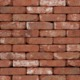•(Edited )

Hi Jonathan,

Various sources (archtoolbox, ballast, etc) will give you the nominal dimensions of modular brick and cmu. These nominal dimensions include the 3/8" mortar joint, so you can divide the area given for a question by the nominal dimension of whatever masonry you are using.

A good number to know is 8".

• Height of 8" CMU
• Length of 1/2 of 8" CMU
• Length of modular brick
• Height of three (3) modular bricks

All of the above dimensions include mortar joints.

So for your example, 1000* sf (50' L x 20' H) of brick requires:

(50' * 12")/8" = 75 bricks for one row

(20' * 12")/8" = 30 three-brick stacks, which is 30*3 = 90 bricks

For the total area, it would be 75 * 90 = 6750 bricks

If the question gives a rate for waste, say 20%, you would then need 6750 * 1.20 = 8100 bricks

Hope this helps,

Anson

* I totally goofed and did the whole example calculation using 1000 sf, not 100sf. Still the method applies.

•(Edited )

Thank you Jonathan for asking this question and thank you Anson to answer this question. It is more complicated than I thought tho. I thought we can just simply use the overall wall area ( let’s say 100sf) divide the brick surface area ( let’s say 2” by 8”) And if it two row then simply multiply by 2. In real life, isn’t that the subcontract can cut the brick to fit the wall?

•Sha Liu,

Your method for quantifying masonry is applicable too.  However, a modular brick course with a typical 3/8" mortar joint does not equal 2"; the height would be measured as 8" / 3.  You would also want to make sure to convert units as necessary.

You are also correct that, in real life, the contractor can cut a brick that is out of coursing if needed.  However, I would think, with NCARB's ideals regarding conservation of materials and resources and wanting to reduce construction waste, any answer option that goes against this line of thinking would probably not be the best possible answer available.